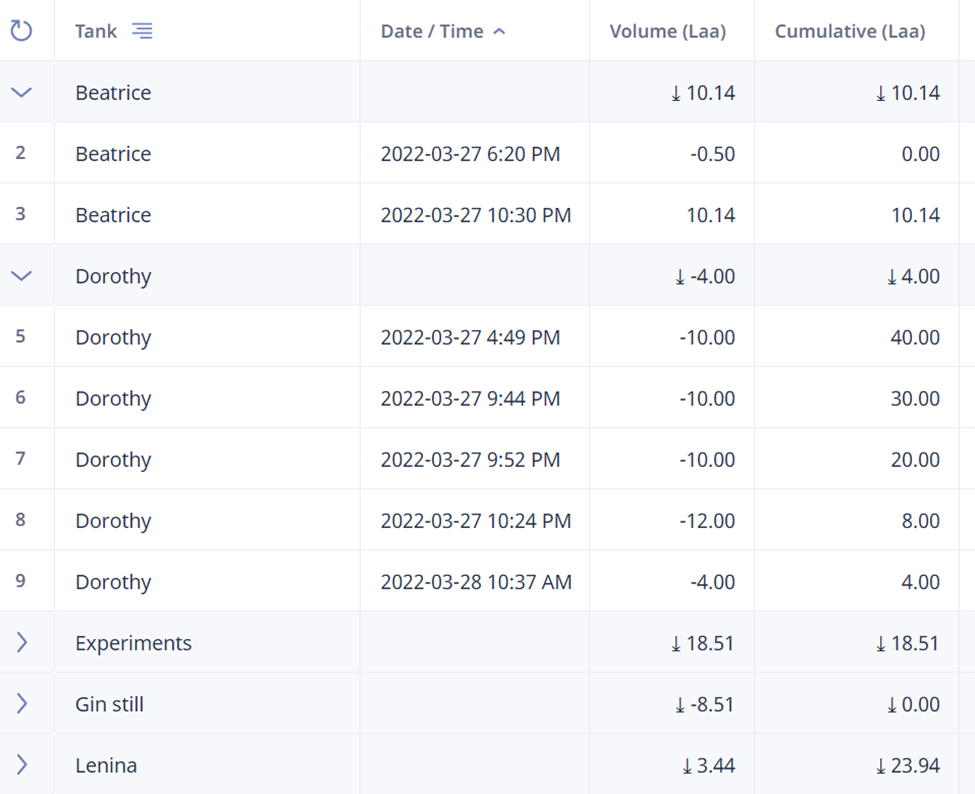0# Finding the sum of the last entry given specific text in a field

Sorry for the nonsensical title.

I have a table called Bulk Inventory:In a formula in a different table, I want to find the sum of the 'Cumulative (Laa)' values for the final entry of a given month for all tanks. So from the screenshot above, I want to add 10.14 plus 4.00 and so on.

I want the formula to work even if I add more tanks in the future.

By the way, loving Ninox so far! Once I have my database set up it's going to save me almost an hour per day on average!

4replies Oldest first
• Oldest first
• Popular•• Lars
• Lars
• 3 mths ago
• Reported - view

Hi Alex,

just summarise all the separate values for one month, i.e. 0.00, 10.14, 40.00, 30.00 ... that will yield the same result and you can easily access these values with a select command on the Tank table and an appropriate month filter. It will go over all the Tanks in the table, no matter how many you'll add or remove

It will be something like

``````sum((select Tank where month(date) = 2).fieldThatYouCumulate)
``````

Does this help?

Like
•• Alex Mumm
• Alex_Mumm
• 3 mths ago
• Reported - view

Lars Unfortunately that doesn't work because there are multiple records for each tank field in this Bulk Inventory table.

Here's what I have so far:

``````let a := this;
let T1 := last(select 'Bulk Inventory' where Tank = "Beatrice" and month(Date) = a.Month).'Cumulative (Laa)';
let T2 := last(select 'Bulk Inventory' where Tank = "Dorothy" and month(Date) = a.Month).'Cumulative (Laa)';
let T3 := last(select 'Bulk Inventory' where Tank = "Experiments" and month(Date) = a.Month).'Cumulative (Laa)';
T1 + T2 + T3
``````

So you can see that currently I need a line of code for each tank (Beatrice, Dorothy, Experiments, etc) and then I add all the values afterwards. Is there a better way to do this?

Like
•• Alex Mumm
• Alex_Mumm
• 3 mths ago
• Reported - view

I think I didn't state my problem clearly enough, but I've found a solution:

``````let a := this;
let b := unique((select 'Bulk Inventory' where month(Date) = a.Month).Tank);
let total := 0;
let i := 0;
while i < count(b) do
total := total + last(select 'Bulk Inventory' where Tank = item(b, i)).'Cumulative (Laa)';
i := i + 1
end
;
total
``````
Like
•• John Halls
• John_Halls
• 3 mths ago
• Reported - view

Hi Alex

Loops like this can be shortened to

``````let a := this;
let total := 0;
for b in unique((select 'Bulk Inventory' where month(Date) = a.Month).Tank) do
total := total + last(select 'Bulk Inventory' where Tank = b).'Cumulative (Laa)';
end;
total
``````

Regards John

Like 2
Like Follow